# Mathematics Quiz For RRB NTPC : 23rd January 2020 for Boat & Stream , Number System And Profit & Loss

Q1. A boat covers 20 km downstream in one hour and covers the same distance upstream in 2 hours then what is the speed of the boat in still water?
(a) 15 km/h
(b) 10 km/h
(c) 5 km/h
(d) 7.5 km/h

Q2. There are some benches in the class. If 4 students sit on each bench then 3 benches remains empty but when 3 students sit on each bench then 3 students could not get their seat. What is the total number of students in the class?
(a) 36
(b) 24
(c) 54
(d) 48

Q3. The sum of seven consecutive numbers is 175. What is the difference between twice of the largest number and thrice of the smallest number?
(a) 8
(b) 7
(c) 10
(d) 12

Q4. A trader invested one-third of his capital in business and half of the rest in share market. If there is Rs. 3,000 left with him then the total capital of the trader is
(a) Rs. 12000
(b) Rs. 9000
(c) Rs. 18000
(d) Rs. 15000

Q5.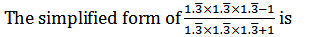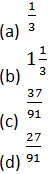6. The largest number among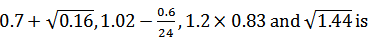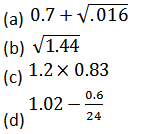Q7.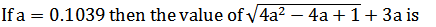(a) 0.1039
(b) 0.2078
(c) 1.1039
(d) 2.1039

Q8.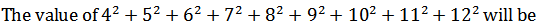(a) 636
(b) 650
(c) 664
(d) 626

Q9. A sum of Rs 20000 becomes Rs 32000 in 12 years, when invested in a scheme of simple interest. If the same sum is invested in a scheme of compound interest with same yearly interest rate (compounding of interest is done yearly), then what will be the amount (in Rs) after 2 years?
(a) 21750
(b) 22050
(c) 23250
(d) 24650

10. A shopkeeper allows an extra discount of 12% on a radio after giving an initial discount of 20%. If the final selling price of the ratio is Rs. 704 then what is the marked price?
(a) Rs. 1000
(b) Rs. 1200
(c) Rs. 800
(d) Rs. 960

Solutions: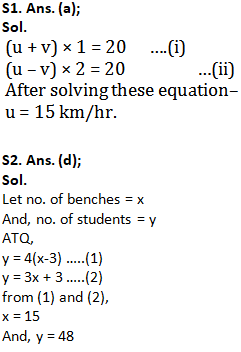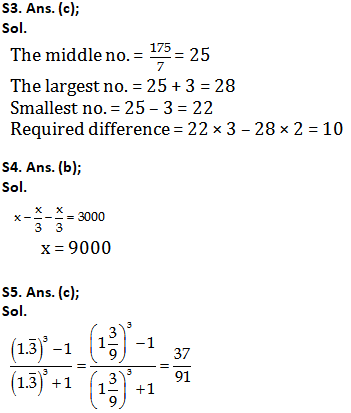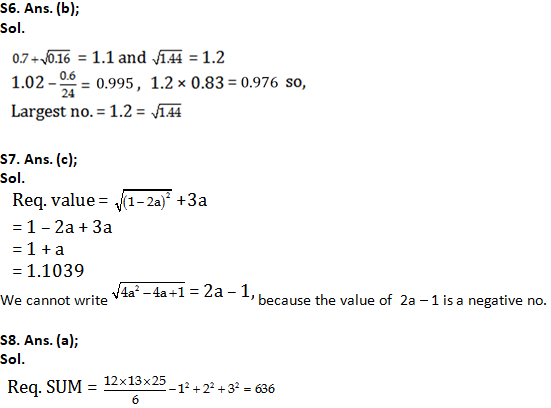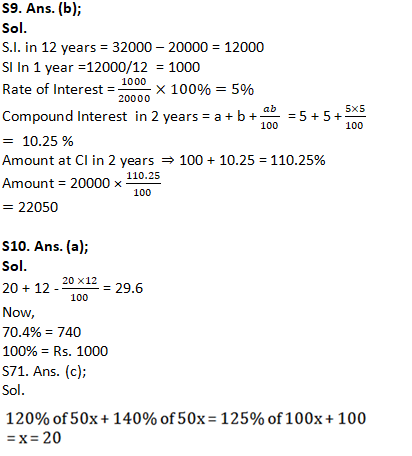SSC CGL | SSC CHSL 2020 | Free Marathon Class English, Science, Maths, Reasoning

Important Links for RRB NTPC Recruitment 2019